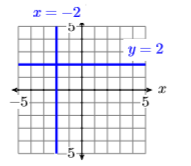# 7.5: Standard Form of a Line

$$\newcommand{\vecs}{\overset { \rightharpoonup} {\mathbf{#1}} }$$ $$\newcommand{\vecd}{\overset{-\!-\!\rightharpoonup}{\vphantom{a}\smash {#1}}}$$$$\newcommand{\id}{\mathrm{id}}$$ $$\newcommand{\Span}{\mathrm{span}}$$ $$\newcommand{\kernel}{\mathrm{null}\,}$$ $$\newcommand{\range}{\mathrm{range}\,}$$ $$\newcommand{\RealPart}{\mathrm{Re}}$$ $$\newcommand{\ImaginaryPart}{\mathrm{Im}}$$ $$\newcommand{\Argument}{\mathrm{Arg}}$$ $$\newcommand{\norm}{\| #1 \|}$$ $$\newcommand{\inner}{\langle #1, #2 \rangle}$$ $$\newcommand{\Span}{\mathrm{span}}$$ $$\newcommand{\id}{\mathrm{id}}$$ $$\newcommand{\Span}{\mathrm{span}}$$ $$\newcommand{\kernel}{\mathrm{null}\,}$$ $$\newcommand{\range}{\mathrm{range}\,}$$ $$\newcommand{\RealPart}{\mathrm{Re}}$$ $$\newcommand{\ImaginaryPart}{\mathrm{Im}}$$ $$\newcommand{\Argument}{\mathrm{Arg}}$$ $$\newcommand{\norm}{\| #1 \|}$$ $$\newcommand{\inner}{\langle #1, #2 \rangle}$$ $$\newcommand{\Span}{\mathrm{span}}$$$$\newcommand{\AA}{\unicode[.8,0]{x212B}}$$

In this section we will investigate the standard form of a line. Let’s begin with a simple example.

Example $$\PageIndex{1}$$

Solve the equation $$2x +3y = 6$$ for $$y$$ and plot the result.

Solution

First we solve the equation $$2x +3y = 6$$ for $$y$$. Begin by isolating all terms containing y on one side of the equation, moving or keeping all the remaining terms on the other side of the equation.

\begin{aligned} 2x+3y &= 6 \quad \color{Red} \text { Original equation. } \\ 2x+3y-2x &= 6-2x \quad \color{Red} \text { Subtract } 2x \text { from both sides. } \\ 3y &= 6-2x \quad \color{Red} \text { Simplify. } \\ \dfrac{3y}{3} &= \dfrac{6-2x}{3} \quad \color{Red} \text { Divide both sides by } 3 \end{aligned} \nonumber

Note

Just as multiplication is distributive with respect to addition $a(b + c)=ab + ac \nonumber$so too is division distributive with respect to addition.$\dfrac{a+b}{c}=\dfrac{a}{c}+\dfrac{b}{c} \nonumber$

When dividing a sum or a diﬀerence by a number, we use the distributive property and divide both terms by that number.

\begin{aligned} y &= \dfrac{6}{3}-\dfrac{2x}{3} \quad \color{Red} \text { On the left, simplify. On the right, divide both terms by } 3 \\ y &= 2-\dfrac{2 x}{3} \quad \color{Red} \text { Simplify. } \end{aligned} \nonumber

Finally, use the commutative property to switch the order of the terms on the right-hand side of the last result.

\begin{aligned} y &= 2+\left(-\dfrac{2 x}{3}\right) \quad \color{Red} \text { Add the opposite. } \\ y &= -\dfrac{2}{3} x+2 \quad \color{Red} \text { Use the commutative property to switch the order. } \end{aligned} \nonumber

Because the equation $$2x +3y = 6$$ is equivalent to the equation $$y=-\dfrac{2}{3} x+2$$, the graph of $$2x +3 y = 6$$ is a line, having slope $$m = −2/3$$ and $$y$$-intercept $$(0 ,2)$$. Therefore, to draw the graph of $$2x+3y = 6$$, plot they-intercept $$(0,2)$$, move down $$2$$ and $$3$$ to the right, then draw the line (see Figure $$\PageIndex{1}$$ ).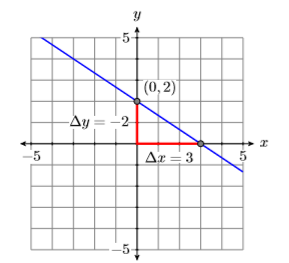Figure $$\PageIndex{1}$$: The graph of $$2x +3y = 6$$, or equivalently, $$y=-\dfrac{2}{3} x+2$$

Exercise $$\PageIndex{1}$$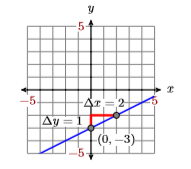In general, unless $$B = 0$$, we can always solve the equation $$Ax + By = C$$ for y:

\begin{aligned} Ax+By &= C \quad \color{Red} \text { Original equation. } \\ Ax+By-Ax &= C-Ax \quad \color{Red} \text { Subtract } Ax \text { from both sides. } \\ By &= C-Ax \quad \color{Red} \text { Simplify. } \\ \dfrac{By}{B} &= \dfrac{C-Ax}{B} \quad \color{Red} \text { Divide both sides by } B \\ y &= \dfrac{C}{B}-\dfrac{Ax}{B} \quad \color{Red} \text { distribute the } B \\ y &= -\dfrac{A}{B}x+\dfrac{C}{B} \quad \color{Red} \text { Commutative property } \end{aligned} \nonumber

Note that the last result is in slope-intercept form $$y = mx+b$$, whose graph is a line. We have established the following result.

FAct

The graph of the equation $$Ax + By = C$$, is a line.

Important points: A couple of important comments are in order.

1. The form $$Ax + By = C$$ requires that the coeﬃcients $$A$$, $$B$$, and $$C$$ are integers. So, for example, we would clear the fractions from the form $\dfrac{1}{2} x+\dfrac{2}{3} y=\dfrac{1}{4} \nonumber$by multiplying both sides by the least common denominator. \begin{aligned} 12\left(\dfrac{1}{2} x+\dfrac{2}{3} y\right) &=\left(\dfrac{1}{4}\right) 12 \\ 6 x+8 y &=3 \end{aligned}\nonumberNote that the coeﬃcients are now integers.
2. The form $$Ax + By = C$$ also requires that the ﬁrst coeﬃcient $$A$$ is nonnegative; i.e., $$A ≥0$$. Thus, if we have $-5 x+2 y=6 \nonumber$then we would multiply both sides by $$−1$$, arriving at: \begin{aligned}-1(-5 x+2 y) &=(6)(-1) \\ 5 x-2 y &=-6 \end{aligned} \nonumberNote that $$A = 5$$ is now greater than or equal to zero.
3. If $$A$$, $$B$$, and $$C$$ have a common divisor greater than $$1$$, it is recommended that we divide both sides by the common divisor, thus “reducing” the coeﬃcients. For example, if we have $3x + 12y =−24 \nonumber$then dividing both side by $$3$$ “reduces” the size of the coeﬃcients.\begin{aligned} \dfrac{3 x+12 y}{3} &=\dfrac{-24}{3} \\ x+4 y &=-8 \end{aligned} \nonumber

Standard form

The form $$Ax + By = C$$, where $$A$$, $$B$$, and $$C$$ are integers, and $$A ≥ 0$$, is called the standard form of a line.

## Slope-Intercept to Standard Form

We’ve already transformed a couple of equations in standard form into slopeintercept form. Let’s reverse the process and place an equation in slope intercept form into standard form.

Example $$\PageIndex{2}$$

Given the graph of the line in Figure $$\PageIndex{2}$$, ﬁnd the equation Given the graph of the line below, ﬁnd the equation of the line in standard form.Figure $$\PageIndex{2}$$: Determine the equation of the line.

Solution

The line intercepts the $$y$$-axis at $$(0,−3)$$. From $$(0,−3)$$, move up $$5$$ units, then left $$2$$ units. Thus, the line has slope $$\Delta y / \Delta x=-5 / 2$$ (see Figure $$\PageIndex{3}$$). Substitute $$−5/2$$ form and $$−3$$ for $$b$$ in the slope-intercept form of the line.Figure $$\PageIndex{3}$$: The line has $$y$$-intercept $$(0,−3)$$ and slope $$−5/2$$.

\begin{aligned} y &= mx+b \quad \color{Red} \text { Slope-intercept form. } \\ y &= -\dfrac{5}{2} x-3 \quad \color{Red} \text { Substitute: }-5 / 2 \text { for } m,-3 \text { for } b \end{aligned} \nonumber

To put this result in standard form $$Ax + By = C$$, ﬁrst clear the fractions by multiplying both sides by the common denominator.

\begin{aligned} 2y &= 2\left[-\dfrac{5}{2} x-3\right] \quad \color{Red} \text { Multiply both sides by } 2 \\ 2y &= 2\left[-\dfrac{5}{2} x\right]-2 \quad \color{Red} \text { Distribute the } 2 \\ 2y &= -5x-6 \quad \color{Red} \text { Multiply. } \end{aligned} \nonumber

That clears the fractions. To put this last result in the form $$Ax+By = C$$, we need to move the term $$−5x$$ to the other side of the equation.

\begin{aligned} 5x+2y &= -5x-6+5x \quad \color{Red} \text { Add } 5x \text { to both sides. } \\ 5x+2y &= -6 \quad \color{Red}\text { Simplify. } \end{aligned} \nonumber

Thus, the standard form of the line is $$5x +2y = −6$$. Note that all the coeﬃcients are integers and the terms are arranged in the order $$Ax+By = C$$, with $$A ≥0$$.

Exercise $$\PageIndex{2}$$

Given the graph of the line below, ﬁnd the equation of the line in standard form.$$3 x−4y = −2$$

## Point-Slope to Standard Form

Let’s do an example where we have to put the point-slope form of a line in standard form.

Example $$\PageIndex{3}$$

Sketch the line passing through the points $$(−3,−4)$$ and $$(1,2)$$, then ﬁnd the equation of the line in standard form.

Solution

Plot the points (−3,−4) and (1,2), then draw a line through them (see Figure $$\PageIndex{4}$$).Figure $$\PageIndex{4}$$: The line through $$(−3,−4)$$ and $$(1,2)$$.

Use the points $$(−3,−4)$$ and $$(1,2)$$ to calculate the slope.

\begin{aligned} \text { Slope } &= \dfrac{\Delta y}{\Delta x} \quad \color{Red} \text { Slope formula. } \\ &= \dfrac{2-(-4)}{1-(-3)} \quad \color{Red} \text { Subtract coordinates of }(-3,-4) \\ &= \dfrac{6}{4} \quad \text { Simplify. } \\ &= \dfrac{3}{2} \quad \text { Reduce. } \end{aligned} \nonumber

Let’s substitute $$\left(x_{0}, y_{0}\right)=(1,2)$$ and $$m =3 /2$$ in the point-slope form of the line. (Note: Substituting $$\left(x_{0}, y_{0}\right)=(-3,-4)$$ and $$m =3 /2$$ would yield the same answer.)

\begin{aligned} y-y_{0} &= m\left(x-x_{0}\right) \quad \color{Red} \text { Point-slope form. } \\ y-2 &= \dfrac{3}{2}(x-1) \quad \color{Red} \text { Substitute: } 3 / 2 \text { for } m, 1 \text { for } x_{0} \end{aligned} \nonumber

The question requests that our ﬁnal answer be presented in standard form. First we clear the fractions.

\begin{aligned} y-2 &= \dfrac{3}{2} x-\dfrac{3}{2} \quad \color{Red} \text { Distribute the } 3 / 2 \\ 2[y-2] &= 2\left[\dfrac{3}{2} x-\dfrac{3}{2}\right] \quad \color{Red} \text { Multiply both sides by } 2 \\ 2y-2 &= 2\left[\dfrac{3}{2} x\right]-2\left[\dfrac{3}{2}\right] \quad \color{Red} \text { Distribute the } 2 \\ 2y-4 &= 3 x-3 \quad \color{Red} \text { Multiply. } \end{aligned} \nonumber

Now that we’ve cleared the fractions, we must order the terms in the form $$Ax+By = C$$. We need to move the term $$3x$$ to the other side of the equation.

\begin{aligned} 2y-4-3x &= 3x-3-3x \quad \color{Red} \text { Subtract } 3 x \text { from both sides. } \\ -3x+2y-4 &= -3 \quad \color{Red} \text { Simplify, changing the order on the left-hand side. } \end{aligned} \nonumber

To put this in the form $$Ax + By = C$$, we need to move the term $$−4$$ to the other side of the equation.

\begin{aligned} -3x+2y-4+4 &= -3+4 \quad \color{Red} \text { Add } 4 \text { to both sides. } \\ -3x+2y &= 1 \quad \color{Red} \text { Simplify. } \end{aligned} \nonumber

It appears that $$−3x+2y = 1$$ is in the form $$Ax +By = C$$. However, standard form requires that $$A ≥ 0$$. We have $$A = −3$$. To ﬁx this, we multiply both sides by $$−1$$.

\begin{aligned} -1[-3x+2y] &= -1 \quad \color{Red} \text { Multiply both sides by }-1 \\ 3x-2y &= -1 \quad \color{Red} \text { Distribute the }-1 \end{aligned} \nonumber

Thus, the equation of the line in standard form is $$3x−2y =−1$$.

Note

If we fail to reduce the slope to lowest terms, then the equation of the line would be: $y-2=\dfrac{6}{4}(x-1) \nonumber$

Multiplying both sides by $$4$$ would give us the result $4y−8=6x−6 \nonumber$or equivalently: $−6x +4y =2 \nonumber$

This doesn’t look like the same answer, but if we divide both sides by $$−2$$, we do get the same result. $3x−2y = −1 \nonumber$

This shows the importance of requiring $$A ≥ 0$$ and “reducing” the coeﬃcients $$A$$, $$B$$, and $$C$$. It allows us to compare our answer with our colleagues or the answers presented in this textbook.

Exercise $$\PageIndex{3}$$

Find the standard form of the equation of the line that passes through the points $$(−2,4)$$ and $$(3,−3)$$.

$$7x +5y =6$$

## Intercepts

We’ve studied the $$y$$-intercept, the point where the graph crosses the $$y$$-axis, but equally important are the $$x$$-intercepts, the points where the graph crosses the $$x$$-axis.Figure $$\PageIndex{5}$$: Each $$x$$-intercept has a $$y$$-coordinate equal to zero.

In Figure $$\PageIndex{5}$$, the graph crosses the $$x$$-axis three times. Each of these crossing points is called an $$x$$-intercept. Note that each of these $$x$$-intercepts has a $$y$$-coordinate equal to zero. This leads to the following rule.

$$x$$ Intercepts

To ﬁnd the $$x$$-intercepts of the graph of an equation, substitute $$y = 0$$ into the equation and solve for $$x$$.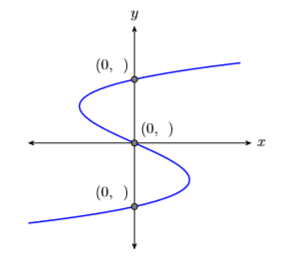Figure $$\PageIndex{6}$$: Each $$y$$-intercept has an $$x$$-coordinate equal to zero.

Similarly, the graph in Figure $$\PageIndex{6}$$ crosses the $$y$$-axis three times. Each of these crossing points is called a $$y$$-intercept. Note that each of these $$y$$-intercepts has an $$x$$-coordinate equal to zero. This leads to the following rule.

$$y$$ Intercepts

To ﬁnd the $$y$$-intercepts of the graph of an equation, substitute $$x = 0$$ into the equation and solve for $$y$$.

Let’s put these rules for ﬁnding intercepts to work.

Example $$\PageIndex{4}$$

Find the $$x$$- and $$y$$-intercepts of the line having equation $$2x−3y = 6$$. Plot the intercepts and draw the line.

Solution

We know that the graph of $$2x−3y = 6$$ is a line. Furthermore, two points completely determine a line. This means that we need only plot the $$x$$- and $$y$$-intercepts, then draw a line through them.

To ﬁnd the $$x$$-intercept of $$2x−3y = 6$$, substitute $$0$$ for $$y$$ and solve for $$x$$.

\begin{aligned} 2 x-3 y &=6 \\ 2 x-3(0) &=6 \\ 2 x &=6 \\ \dfrac{2 x}{2} &=\dfrac{6}{2} \\ x &=3 \end{aligned} \nonumber

Thus, the $$x$$-intercept of the line is $$(3,0)$$.

To ﬁnd the $$y$$-intercept of $$2x−3y = 6$$, substitute $$0$$ for $$x$$ and solve for $$y$$.

\begin{aligned} 2 x-3 y &=6 \\ 2(0)-3 y &=6 \\-3 y &=6 \\ \frac{-3 y}{-3} &=\frac{6}{-3} \\ y &=-2 \end{aligned} \nonumber

Thus, the $$y$$-intercept of the line is $$(0,−2)$$.

Plot the $$x$$-intercept $$(3,0)$$ and the $$y$$-intercept $$(0,−2)$$ and draw a line through them (see Figure $$\PageIndex{7}$$).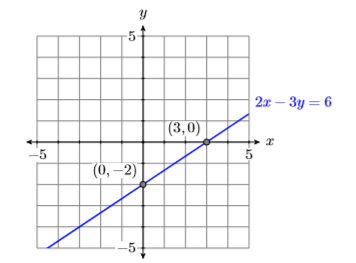Figure $$\PageIndex{7}$$: The graph of $$2x−3y = 6$$ has intercepts $$(3,0)$$ and $$(0,−2)$$.

Exercise $$\PageIndex{4}$$

Find the $$x$$- and $$y$$-intercepts of the line having equation $$3x +4y = −12$$. Plot the intercepts and draw the line.

$$x$$-intercept: $$(−4,0)$$

$$y$$-intercept: $$(0,−3)$$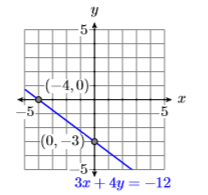Example $$\PageIndex{5}$$

Sketch the line $$4x +3 y = 12$$, then sketch the line through the point $$(−2,−2)$$ that is perpendicular to the line $$4x +3 y = 12$$. Find the equation of this perpendicular line.

Solution

Let’s ﬁrst ﬁnd the $$x$$- and $$y$$-intercepts of the line $$4x +3y = 12$$.

To ﬁnd the $$x$$-intercept of the line $$4x+3y = 12$$, substitute $$0$$ for $$y$$ and solve for $$x$$.

\begin{aligned} 4 x+3 y &=12 \\ 4 x+3(0) &=12 \\ 4 x &=12 \\ \dfrac{4 x}{4} &=\dfrac{12}{4} \\ x &=3 \end{aligned} \nonumber

Thus, the $$x$$-intercept of the line is $$(3,0)$$.

To ﬁnd the $$y$$-intercept of the line $$4x+3y = 12$$, substitute $$0$$ for $$x$$ and solve for $$y$$.

\begin{aligned} 4 x+3 y &=12 \\ 4(0)+3 y &=12 \\ 3 y &=12 \\ \dfrac{3 y}{3} &=\dfrac{12}{3} \\ y &=4 \end{aligned} \nonumber

Thus, the $$y$$-intercept of the line is $$(0,4)$$.

Plot the intercepts and draw a line through them. Note that it is clear from the graph that the slope of the line $$3x +4y = 12$$ is $$−4/3$$ (see Figure $$\PageIndex{8}$$).Figure $$\PageIndex{8}$$: The graph of $$4x+3y = 12$$ has intercepts $$(3,0)$$ and $$(0,4)$$ and slope $$−4/3$$.

You could also solve for $$y$$ to put $$3x +4 y = 12$$ in slope intercept form in order to determine the slope.

Because the slope of $$3x+4y = 12$$ is $$−4/3$$, the slope of a line perpendicular to $$3x +4y = 12$$ will be the negative reciprocal of $$−4/3$$, namely $$3/4$$. Our perpendicular line has to pass through the point $$(−2,−2)$$. Start at $$(−2,−2)$$, move $$3$$ units upward, then $$4$$ units to the right, then draw the line. It should appear to be perpendicular to the line $$3x +4y = 12$$ (see Figure $$\PageIndex{9}$$).Figure $$\PageIndex{9}$$: The slope of the perpendicular line is the negative reciprocal of $$−4/3$$, namely $$3/4$$.

Finally, use the point-slope form, $$m =3 /4$$, and $$\left(x_{0}, y_{0}\right)=(-2,-2)$$ to determine the equation of the perpendicular line.

\begin{aligned} y-y_{0} &= m\left(x-x_{0}\right) \quad \color{Red} \text { Point-slope form. } \\ y-(-2) &= \dfrac{3}{4}(x-(-2)) \quad \color{Red} \text { Substitute: } 3 / 4 \text { for } m,-2 \text { for } x_{0} \text { and }-2 \text { for } y_{0} \\ y+2 &= \dfrac{3}{4}(x+2) \quad \color{Red} \text { Simplify. } \end{aligned} \nonumber

Let’s place our answer in standard form. Clear the fractions.

\begin{aligned} y+2 &= \dfrac{3}{4} x+\dfrac{6}{4} \quad \color{Red} \text { Distribute } 3 / 4 \\ 4[y+2] &= 4\left[\dfrac{3}{4} x+\dfrac{6}{4}\right] \quad \color{Red} \text { Multiply both sides by } 4 \\ 4y+4 &= 4\left[\dfrac{3}{4} x\right]+4\left[\dfrac{6}{4}\right] \quad \color{Red} \text { Distribute the } 4 \\ 4y+8 &= 3x+6 \quad \color{Red} \text { Multiply. } \end{aligned} \nonumber

Rearrange the terms to put them in the order $$Ax + By = C$$.

\begin{aligned} 4y+8-3x &= 3x+6-3x \quad \color{Red} \text { Subtract } 3x \text { from both sides. } \\ -3x+4y+8 &= 6 \quad \color{Red} \text { Simplify. Rearrange on the left. } \\ -3x+4y+8-8 &= 6-8 \quad \color{Red} \text { Subtract } 8 \text { from both sides. } \\ -3x+4y &= -2 \quad \color{Red} \text { Simplify. } \\ -1(-3x+4y) &= -1(-2) \quad \color{Red} \text { Multiply both sides by }-1 \\ 3x-4y &= 2 \quad \color{Red} \text { Distribute the }-1 \end{aligned} \nonumber

Hence, the equation of the perpendicular line is $$3x−4y = 2$$.

Exercise $$\PageIndex{5}$$

Find the equation of the line that passes through the point $$(3,2)$$ and is perpendicular to the line $$6x−5y = 15$$.

$$5x +6y = 27$$

## Horizontal and Vertical Lines

Here we keep an earlier promise to address what happens to the standard form $$Ax + By = C$$ when either $$A = 0$$ or $$B = 0$$. For example, the form $$3x = 6$$, when compared with the standard form $$Ax + By = C$$, has $$B = 0$$. Similarly, the form $$2y = −12$$, when compared with the standard form $$Ax + By = C$$, has $$A = 0$$. Of course, $$3 x = 6$$ can be simpliﬁed to $$x = 2$$ and $$2 y = −12$$ can be simpliﬁed to $$y = −6$$. Thus, if either $$A = 0$$ or $$B = 0$$, the standard form Ax + By = C takes the form $$x = a$$ and $$y = b$$, respectively.

As we will see in the next example, the form $$x = a$$ produces a vertical line, while the form $$y = b$$ produces a horizontal line.

Example $$\PageIndex{6}$$

Sketch the graphs of $$x = 3$$ and $$y = −3$$.

Solution

To sketch the graph of $$x = 3$$, recall that the graph of an equation is the set of all points that satisfy the equation. Hence, to draw the graph of $$x = 3$$, we must plot all of the points that satisfy the equation $$x = 3$$; that is, we must plot all of the points that have an $$x$$-coordinate equal to $$3$$. The result is shown in Figure $$\PageIndex{10}$$.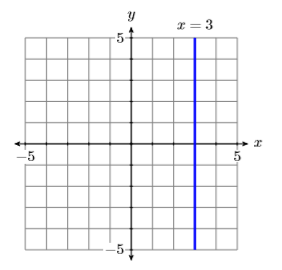Figure $$\PageIndex{10}$$: The graph of $$x = 3$$ is a vertical line.

Secondly, to sketch the graph of $$y = −3$$, we plot all points having a $$y$$-coordinate equal to $$−3$$. The result is shown in Figure $$\PageIndex{11}$$.Figure $$\PageIndex{11}$$: The graph of $$y =−3$$ is a horizontal line.

Things to note:

A couple of comments are in order regarding the lines in Figures $$\PageIndex{10}$$ and $$\PageIndex{11}$$.

1. The graph of $$x = 3$$ in Figure $$\PageIndex{10}$$, being a vertical line, has undeﬁned slope. Therefore, we cannot use either of the formulae $$y = mx + b$$ or $$y−y_0 = m(x−x_0)$$ to obtain the equation of the line. The only way we can obtain the equation is to note that the line is the set of all points $$(x,y)$$ whose $$x$$-coordinate equals $$3$$.
2. However, the graph of $$y = −3$$, being a horizontal line, has slope zero, so we can use the slope-intercept form to ﬁnd the equation of the line. Note that the $$y$$-intercept of this graph is $$(0,−3)$$. If we substitute these numbers into $$y = mx + b$$, we get:\begin{aligned} y &= mx+b \quad \color{Red} \text { Slope-intercept form. } \\ y &= 0x+(-3) \quad \color{Red} \text { Substitute: } 0 \text { for } m,-3 \text { for } b \\ y &= -3 \quad \color{Red} \text { Simplify. } \end{aligned} \nonumber

However, it is far easier to just look at the line in Figures $$\PageIndex{11}$$ and note that it is the collection of all points $$(x,y)$$ with $$y = 3$$.

Exercise $$\PageIndex{6}$$

Sketch the graphs of $$x = −2$$ and $$y = 2$$.